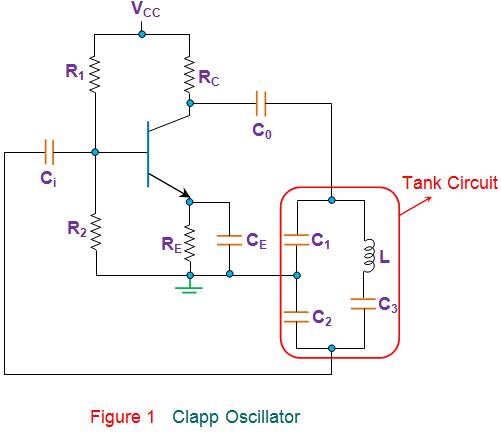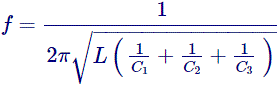# Clapp Oscillator: Frequency Formula And Circuit Diagram

## What is a Clapp Oscillator?

A Clapp oscillator (also known as a Gouriet oscillator) is an LC electronic oscillator that uses a particular combination of an inductor and three capacitors to set the oscillator’s frequency (see circuit diagram below). LC oscillators use a transistor (or vacuum tube or other gain element) and a positive feedback network.

A Clapp oscillator is a variation of a Colpitts oscillator where an additional capacitor (C3) is added into the tank circuit to be in series with the inductor in it, as shown in the circuit diagram below.Apart from the presence of an extra capacitor, all other components and their connections remain similar to that in the case of Colpitts oscillator.

Hence, the working of this circuit is almost identical to that of the Colpitts, where the feedback ratio governs the generation and sustainity of the oscillations. However the frequency of oscillation in the case of a Clapp oscillator is given byUsually, the value of C3 is chosen to be much smaller than the other two capacitors. This is because, at higher frequencies, the smaller the C3, the larger will be the inductor, which eases the implementation as well as reduces the influence of stray inductance.

Nevertheless, the value of C3 is to be chosen with utmost care. This is because, if it is chosen to be very small, then the oscillations will not be generated as the L-C branch will fail to have a net inductive reactance.

However, here it is to be noted that when C3 is chosen to be smaller in comparison with C1 and C2, the net capacitance governing the circuit will be more dependent on it.

Thus the equation for the frequency can be approximated asFurther, the presence of this extra capacitance will make the Clapp oscillator preferable over Colpitts when there is a need to vary the frequency as is the case with Variable Frequency Oscillator (VCO). The reason behind this can be explained as follows.

In the case of the Colpitts oscillator, the capacitors C1 and C2 need to be varied in order to vary their frequency of operation. However during this process, even the feedback ratio of the oscillator changes which in turn affects its output waveform.

One solution to this problem is to make both C1 and C2 to be fixed in nature while achieving the variation in frequency using a separate variable capacitor.

As could be guessed, this is what the C3 does in the case of the Clapp oscillator, which in turn makes it more stable over Colpitts in terms of frequency.

The frequency stability of the circuit can be even more increased by enclosing the entire circuit in a chamber with constant temperature and by using a Zener diode to ensure constant supply voltage.

In addition, it is to be noted that the values of the capacitors C1 and C2 are prone to the effect of stray capacitances unlike that of C3.

This means that the resonant frequency of the circuit would be affected by the stray capacitances if one had a circuit with just C1 and C2, as in the case of Colpitts oscillator.

However, if there is C3 in the circuit, then the changes in the values of C1 and C2 would not vary the resonant frequency much, as the dominant term would then be C3.

Next, it is seen that the Clapp oscillators are comparatively compact as they employ a relatively small capacitor to tune the oscillator over a wide frequency band. This is because, here, even a slight change in the value of the capacitance varies the frequency of the circuit up to a great extent.

Further, they exhibit a high Q factor with a high L/C ratio and lesser circulating current in comparison with Colpitts oscillators.

Lastly, it is to be noted that these oscillators are highly reliable and are hence preferred in spite of having a limited range of frequency of operation.

Want To Learn Faster? 🎓
Get electrical articles delivered to your inbox every week.
No credit card required—it’s 100% free.